# Difference between revisions of "Gradient descent with exact line search for a quadratic function of multiple variables"

## Setup

This page describes gradient descent with exact line search for a quadratic function of multiple variables. Since the function is quadratic, its restriction to any line is quadratic, and therefore the line search on any line can be implemented using Newton's method. Therefore, the analysis on this page also applies to using gradient descent using Newton's method for a quadratic function of multiple variables.

### Function

The function we are interested is a function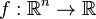$f: \R^n \to \R$ of the form:$f(\vec{x}) = \vec{x}^TA\vec{x} + \vec{b}^T\vec{x} + c$

where$A$ is a$n \times n$ symmetric positive-definite matrix with entries$a_{ij}$ and$\vec{b}$ is the column vector with entries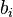$b_i$. For part of this page, we will generalize somewhat to the case that$A$ is a symmetric positive-semidefinite matrix.

Note that we impose the condition of symmetry because we could replace the matrix$A$ with the symmetric matrix$(A + A^T)/2$ without changing the functional form. The positive-definite or positive-semidefinite condition is to guarantee that the function has the right sort of convexity. In the positive-definite case, we are guaranteed that there is a unique point of local minimum that is also the point of absolute minimum. The positive-semidefinite case is somewhat more complicated: we could either have no minimum, or we could have an affine space worth of points at which the function has a local and absolute minimum.

### Learning algorithm

The algorithm starts with a guess$\vec{x}_0$ and updates according to the rule: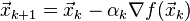$\vec{x}_{k+1} = \vec{x}_k - \alpha_k \nabla f(\vec{x}_k)$

where$\alpha_k$ is the second derivative of$f$ along the direction of the gradient vector. Explicitly, if we set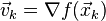$\vec{v}_k = \nabla f (\vec{x}_k)$, we have:$\alpha_k = \frac{|\vec{v}_k|^2}{\vec{v}_k^T(2A)\vec{v}_k}$

where the matrix$2A$ in the denominator arises as the Hessian of the function$f$. Since the function is quadratic, the Hessian is globally constant.

## Convergence properties

We will carry out our analysis of eigenvalues in terms of the Hessian matrix$2A$. If we were instead dealing with the eigenvalues of$A$, we would have to double them to get the eigenvalues of$2A$. Since the bounds on the learning rate are described as reciprocals of the eigenvalues, we would therefore obtain additional factors of 2 in the denominators, or equivalently, we would remove the factor of 2 from the numerators.

Note that in the case$n = 1$, we have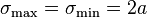$\sigma_{\max} = \sigma_{\min} = 2a$ and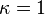$\kappa = 1$ in the notation below.

### Detailed analysis after transforming the problem to a setting where the coordinates are simple

Suppose the eigenvalues of$2A$ are:$\sigma_\max = \sigma_1 \ge \sigma_2 \ge \sigma_3 \ge \dots \ge \sigma_n = \sigma_\min$

Via a coordinate change of the domain using an orthogonal matrix, we can transform$A$ into a diagonal matrix with positive real entries. These entries are halves of the eigenvalues of$2A$. Via a translation, we can get rid of the linear term, and finally, we can translate the function value by a constant (this does not affect the point of local minimum, though it affects the minimum value). We can therefore obtain the following simplified functional form:$f(\vec{x}) = \frac{1}{2} \sum_{i=1}^n \sigma_ix_i^2$

The unique local and absolute minimum is at the zero vector.

Note that even though we know that our matrix can be transformed this way, we do not in general know how to bring it in this form -- if we did, we could directly solve the problem without using gradient descent (this is an alternate solution method). However, even though we may not know the explicit diagonalized form of the function, the fact that it does have such a form gives us information about how the gradient descent process converges.

Starting with a point:$\vec{x} = (x_1,x_2,\dots,x_n)$

we obtain that the gradient vector at the point is:$\nabla f(\vec{x}) = (\sigma_1x_1,\sigma_2x_2,\dots,\sigma_nx_n)$

The multiplier$\alpha$ works out to:$\alpha = \frac{\sum_{j=1}^n \sigma_j^2x_j^2}{\sum_{i=1}^n \sigma_j^3x_j^3}$

Therefore, the update in each coordinate is as follows:$x_i^{\mbox{new}} = x_i - \sigma_i x_i \frac{\sum_{j=1}^n \sigma_j^2x_j^2}{\sum_{i=1}^n \sigma_j^3x_j^3}$

This can also be simplified as:$x_i^{\mbox{new}} = x_i\left(1 - \sigma_i \frac{\sum_{j=1}^n \sigma_j^2x_j^2}{\sum_{i=1}^n \sigma_j^3x_j^3} \right)$